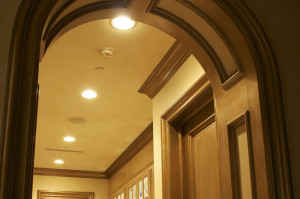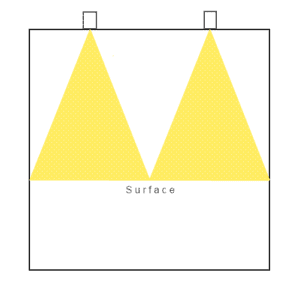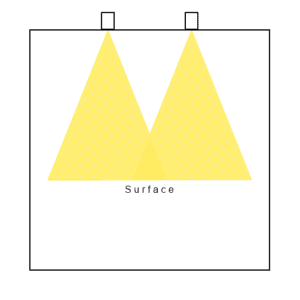# Recessed Lighting SpacingThe formula for spacing recessed lighting in a room is simple but important in terms of appearance and function.

In this short post, I’ll give you the formula and then explain why it provides the ideal distribution of light.

### The Formula

Here’s the formula for proper recessed lighting spacing: The distance between the lights is always double what it is at the ends.

### The Formula Explained

Although the formula above is a fairly simple concept, it’s important to understand because it’s the key to great lighting.

Light shines down from a recessed light fixture in the shape of a cone. In order to provide an even distribution of light, each cone should intersect at the surface you are illuminating.The image to the right is an example of cones of light from a side view.

If you look at the fixtures in the ceiling, you’ll notice that the distance between them is twice as far as the distance from either light to the edge.

Now look at each triangle. If you draw a vertical line through each one, you end up with four half-triangles; one on each end and two in between (or double).A common mistake is to install recessed lighting with equal spacing between the lights and the walls.

When the spacing from the wall to the first light is the same as the spacing between the lights, you end up with bright spots between the lights and dark edges.

Now that you have an understanding of the principle behind spacing recessed lights, you can apply it to calculate recessed lighting placement.